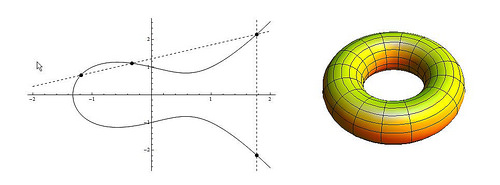## High rank elliptical curves and diophantine tuplesElliptical curves became a central research topic in algebra and number theory. They were not only used to proof the Fermat’s theorem, but also in modern cryptosystems. A range of interesting questions arises analyzing elliptical curves. The algorithmic treatment of these questions are in the focus of many current research projects, especially with the application in the field of data security. Thereby fundamental algorithms of discrete mathematics are used. The students are already confronted with such an algorithm at school, the Euclidean algorithm. The Euclidean algorithm calculates the greatest common divisor of two natural numbers. By trying to expand this algorithm to real numbers the continued fraction algorithm can be obtained, which is especially relevant in the number theory. By using the theory about the continued fractions it is for example possible to describe all solutions of the so called Pell’s equation completely. Despite the age of Pell’s equation, it is a fundamental problem in the current research. This and other algorithms from discrete mathematics provide the basis for the experiments of this research project.
An elliptic curve E over the rational numbers can be equipped with an Abelian group law in a natural way (with the secant-tangent-method), which makes E a finitely generated Abelian group. This Abelian group is for example used in cryptography, especially if the resources (memory, speed) are limited. That’s because the keys used during the crypto-procedures can be chosen much smaller while maintaining the same level of security. To guarantee the safety of the procedure the question arises whether elliptic curves with an arbitrary high rank exist. The assumption is yes. In this project new rank records are to be established. Explicit equations of the elliptic curves are of interest in particular. Referred to the theorem of Mazur there are only 15 explicit known finite groups that are possible for the torsion subgroup. Another aim is to construct elliptic curves with high rank for a given torsion subgroup. Elliptic curves occur with so called Diophantic tupels in a natural way. Starting from polynomial families of Diophantic D(n)-m-tuples new elliptic curves with high rank are constructed in this project. Other questions during this project concerning the Diophantic tupels are:

• Proof of the quintuplet-assumption: There is no Diophantic D(1)-quintuplet.